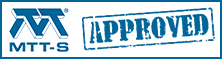# ADS Example 1. Two-state devices

This page will show you how to create a two-state network schematic for use in a larger schematic, using measured S2P data. We assume that the reader has some knowledge of ADS and what a "parameter" means in a schematic.

This technique is useful for any network design that might operate in more than one mode of operation, such an RF switch, or a digital phase shifter or an attenuator. The thing that you are trying to do is provide a single ADS schematic (a network in its own .dsn file) that simulates the response of a single device that could be in one of two states or modes of operation, such as a switch FET measured in its "on" and "off" states (biased at VGS=0V, -5V for example). Note that the ADS network schematic could contain data blocks referencing measured S-parameters, or a switchable equivalent circuit model, or a mix, and it could be a one-port, two-port... or an N-port. Thanks to Leo for helping us clear that up!

The trick we employ is to add a parameter to the schematic, which we call "ON", to which we will later assign either a value of 0, or a value of 1. Below we use this parameter to define a variable resistor that connect thes input and output of a two-port network to one of two different networks defined by the measured S2P files of a switch FET measured in its "on" and "off" states (but we could just as well use it to connect to two different circuit models).

In the schematic below, four two-state resistors are used to connect port 1 and port 2 to either the "FET_ON.s2p" two-port or to the "FET_OFF.s2p" two-port. The top two resistors are controlled by R1, the bottom two resistors are controlled by R2. When R1 is set to zero and R2 is set to infinity, the circuit will behave like a switch-FET that is ON. When R1 is infinity and R2 is zero, it will behave like a switch-FET that is off.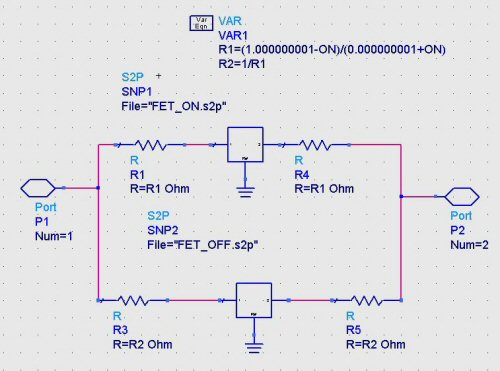The resistors need to be either open or short circuits for the circuit to behave as intended, but the parameter "ON" is either 0, or 1. How do we do make this work? In the "VAR" block we create equations to use the "ON" variable to toggle the resistors from a very small number (close to zero) to a very high number (approaching infinity), with "ON" as the dependent variable:

R1=(1.000000001-ON)/(0.000000001+ON)

R2=1/R1

When ON=0, R1 will be very high (actually, 1,000,000,001) which is close enough to an open circuit to serve our purpose. When ON=1, R1 is very close to zero (0.000,000,001), close enough to a short circuit. Because the value of R2 must be opposite of R1, we merely take the reciprocal of R1 to arrive at R2.

Now let's place the resulting two-port circuit into a new schematic, and set ON=1, and analyze the response: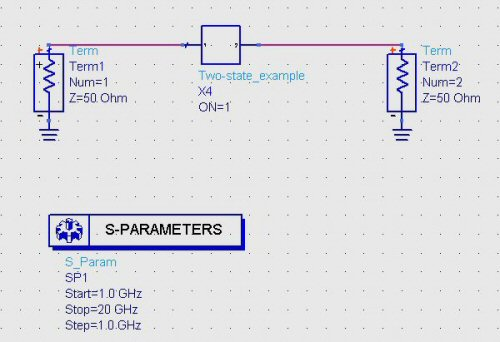Pulling up the S-parameters in a separate window, here we see the ON state of the FET, where S21 is very close to zero dB at DC (a direct connection), because the FET looks like a small-value resistor.

OOPS, graph is missing! but you can look further down at the last graph to see the S21 data until I can recreate the missing graph

Now let's look at the OFF state by setting ON=0: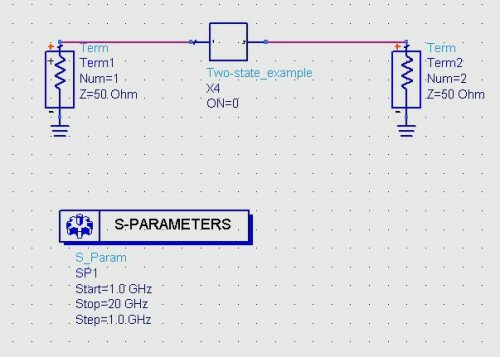Analyzing the circuit, we see the OFF state S-parameters below. In this case S21 is far from zero dB at DC because the FET looks like a series capacitor: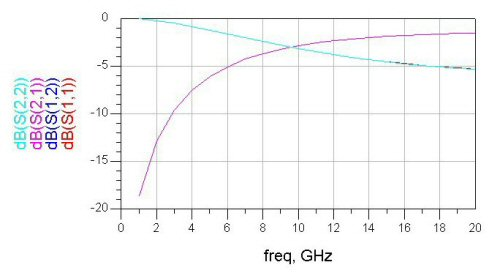How about if you want to compare both states on the same graph? Create a four-port circuit, with the two-state schematic entered twice, once with ON=0, and once with ON=1.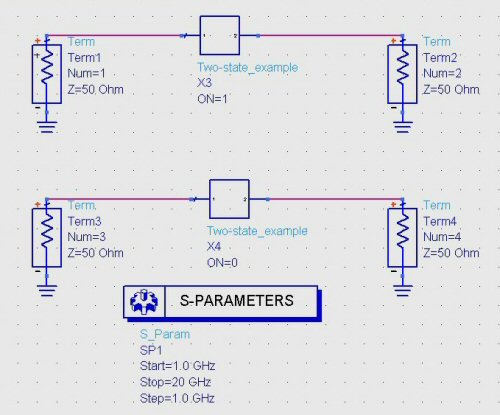Now we can display ON and OFF state transmission coefficients at the same time, as S21 and S43.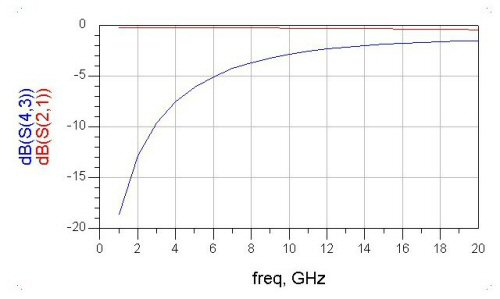Here's an example of a two state device, in this case it's a switch FET where we use an equivalent circuit model, and add parallel inductor to resonate the FET's off capacitance.

We'll post more ADS examples from time to time. Send us an email if you have any suggested topics!

Author : Unknown Editor